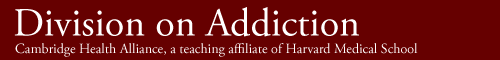Site Contents:

Other Division web sites:

Facing the Odds: The Mathematics of Gambling and Other Risks

The purpose of this curriculum is to enhance students' interest in mathematics and provide the knowledge and skills that can help students to think more critically. This curriculum aims to make mathematics more meaningful to students and more relevant to their daily lives by introducing concepts of probability and statistics through the use of gambling- and media-related topics. With the proliferation of gambling opportunities throughout America, young people have exposure to, and inherent curiosity in gambling-related matters; this curriculum uses this curiosity to present important mathematical concepts. For example, students will learn about randomness and chance as these concepts relate to probability and gambling, the probability of winning the lottery, and the use of statistics in the media and everyday life. This curriculum also will help students to develop critical thinking and number sense skills and to apply these skills to media, advertising, and gambling issues. In particular, students will learn to make decisions and choices about gambling activities based on mathematical reasoning. Contemporary research shows that gambling behavior is prevalent among youth and that a proportion of young people are experiencing problems related to gambling. This curriculum provides an opportunity for students to learn about mathematics through relevant contemporary social issues has the potential to increase student interest in mathematics and concurrently to diminish the likelihood of the development of addictive behaviors.

How To Use This Curriculum

Note: Exercise 1-10 in Section 1 illustrates the concept that with any group of 23 people, there is a 50% chance that two or more people in that group will have the same birthday (Paulos, 1988, p. 27; McGervey, 1986, p. 9).

A teacher wrote:

I taught the "Facing the Odds The Mathematics of Gambling" with my 8th grade class in St. Gabriel, LA. I found a shorter way to solve the "Shared Birthdays" activity in your curriculum. It seems that you will get the same result if you use the following formula: (Permutation of 365 items taken N at a time) divided by (365 raised to the N power). Where N is the number of people in the activity. In an abbreviated for it would look like this: P(365,n)/(365^n). If you find that this formula works, let me know if you'd like to include it in your "Facing the Odds Curriculum." The formula worked for me each time, in place of the long tedious calculation in the curriculum.

The Division Responds:

You are absolutely correct. The combined probabilities in the "Shared Birthday" activity, 364/365 * 363/365 * 362/365 ... can be simplified. The continued product of the common denominator, 365, can be expressed as a power of 365. The numerators can also be expressed as the permutation of 365 items taken N at a time.

However, the simplified expression may not carry the same meaning for the students. One goal of the exercise is to introduce the concept of combined probabilities. The concept that there are 364 days that are not the same as the first birthday, 363 days that are not the same as two non-matching days, etc. retains the idea that the number of possible non-matching days decreases and finding one is increasingly less likely. Perhaps even more powerful is the opportunity to examine the effect of combined probabilities. The last term in the continued product (for N = 23) is 343/365 = .94. In simple terms this is still a large probability and students may be amazed at how quickly the product of what seem to be large probabilities turns into a relatively small probability. Other less computationally-demanding exercises could also be used. For example, you could ask students to figure out how large a group of people would have a greater than 50% chance of having at least two people born on the same day of the week.

The simplification that you pointed out could ease computation but might obscure the "number sense" that derives from going through the longer process. Once the concept of combinatorial probabilities is learned, simplifying the computation could be a valuable opportunity to introduce or revisit concepts such as permutation that go into the simplification. I would not move too quickly to the simplification for fear that the basic goal of the exercise would not be met.

Richard A. LaBrie, Ed.D.
Associate Director of Research and Data Analysis
Harvard Medical School Division on Addictions